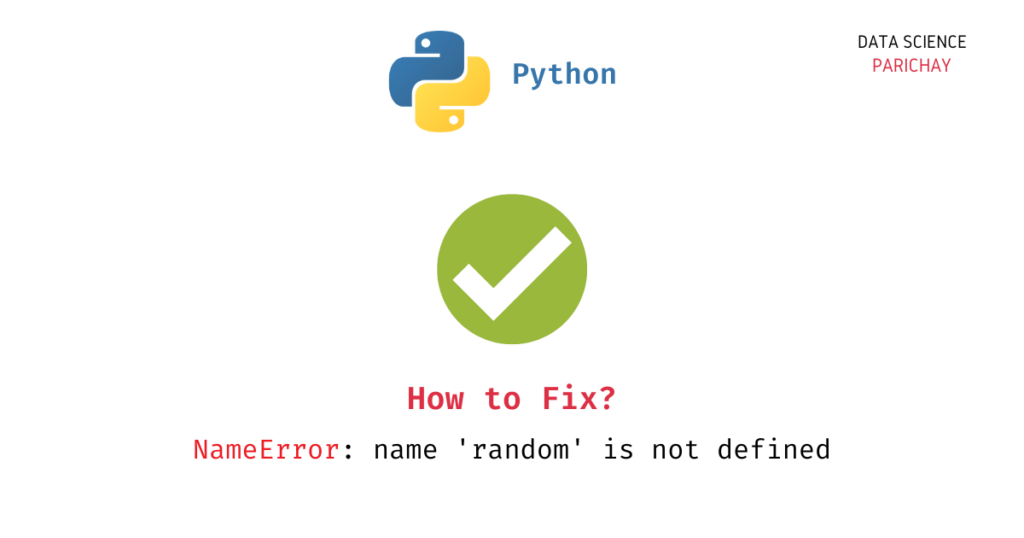# How to Fix – NameError: name ‘random’ is not defined

If you are working with Python and trying to use the random library, you may encounter the “NameError: name ‘random’ is not defined” error. In this tutorial, we will explore why this error occurs and the steps required to fix it such that your Python code can successfully run without errors.

We will cover common causes of the error and provide solutions to help you get your code up and running quickly. So, let’s get started!

## Why does the `NameError: name 'random' is not defined` error occur?

This error occurs when you try to use the random library in your Python code, but Python cannot find the random module in its namespace. The following are some of the scenarios in which this error usually occurs.

1. You have not imported the random module.
2. You have imported the random module using a different name.

## How to fix the `NameError: name 'random' is not defined`?

The `random` library in Python is a built-in module that provides a suite of functions for generating random numbers. It can be used to generate random integers, floating-point numbers, and sequences such as lists and tuples. It is commonly used in games, simulations, and statistical analysis. Since this library is a built-in library in Python, you don’t need to separately install it. You can import it and start using it.

Let’s now look at the above scenarios in detail.

### The `random` module is not imported

It can happen that you are trying to use the `random` module without even importing it. This is because Python does not recognize the random library and its functions until it is imported into the code.

For example, let’s try to use the `random` module without importing it and see what we get.

```# note that random is not imported

# generate a random integer between 1 and 10
print(random.randint(1, 10))```

Output:

📚 Data Science Programs By Skill Level

Introductory

Intermediate ⭐⭐⭐

🔎 Find Data Science Programs 👨‍💻 111,889 already enrolled

Disclaimer: Data Science Parichay is reader supported. When you purchase a course through a link on this site, we may earn a small commission at no additional cost to you. Earned commissions help support this website and its team of writers.

```---------------------------------------------------------------------------

NameError                                 Traceback (most recent call last)

Cell In, line 4
1 # note that random is not imported
2
3 # generate a random integer between 1 and 10
----> 4 print(random.randint(1, 10))

NameError: name 'random' is not defined```

We get a `NameError` stating that the name `random` is not defined. To use the `random` library, you need to import it first.

```import random

# generate a random integer between 1 and 10
print(random.randint(1, 10))```

Output:

`4`

Here, we are importing the `random` module first and then using it to generate a random integer between 1 and 10. You can see that we did not get any errors here.

You can also get a `NameError` if you are importing only specific parts of the library and then trying to access the entire `random` library. For example –

```from random import randint

# generate a random integer between 1 and 10
print(random.randint(1, 10))```

Output:

```---------------------------------------------------------------------------

NameError                                 Traceback (most recent call last)

Cell In, line 4
1 from random import randint
3 # generate a random integer between 1 and 10
----> 4 print(random.randint(1, 10))

NameError: name 'random' is not defined```

We get a `NameError` here because we are importing only the `randint()` function from the `random` library but we are trying to access the entire library. To resolve the above error, either only use the specific method imported or import the `random` library altogether.

### The `random` module is imported using a different name

If you import the random module using a different name, for example `import random as rnd`, and then try to use the name “random” to use it, you will get a `NameError` because the name “random” is not defined in your current namespace.

Let’s look at an example.

```import random as rnd

# generate a random integer between 1 and 10
print(random.randint(1, 10))```

Output:

```---------------------------------------------------------------------------

NameError                                 Traceback (most recent call last)

Cell In, line 4
1 import random as rnd
3 # generate a random integer between 1 and 10
----> 4 print(random.randint(1, 10))

NameError: name 'random' is not defined```

We get a `NameError: name 'random' is not defined`. This is because we have imported the `random` module with the name `rnd` but we’re trying to use it using the name `random`.

To fix this error, you can either access random using the name that you have used in the import statement or import random without an alias.

```import random as rnd

# generate a random integer between 1 and 10
print(rnd.randint(1, 10))```

Output:

`9`

In the above example, we are importing `random` as `rnd` and then using `rnd` to access the `random` module’s methods.

Alternatively, as seen in the example in the previous section, you can import `random` without any aliases and simply use `random` to avoid the `NameError`.

## Conclusion

In conclusion, encountering a `NameError: name 'random' is not defined` error can be frustrating, but it is a common issue that can be easily fixed. By ensuring that the `random` module is imported correctly and that the correct syntax is used when calling its functions, you can avoid this error and successfully execute your code.

You might also be interested in –

## Author

•Piyush is a data professional passionate about using data to understand things better and make informed decisions. He has experience working as a Data Scientist in the consulting domain and holds an engineering degree from IIT Roorkee. His hobbies include watching cricket, reading, and working on side projects.

Scroll to Top